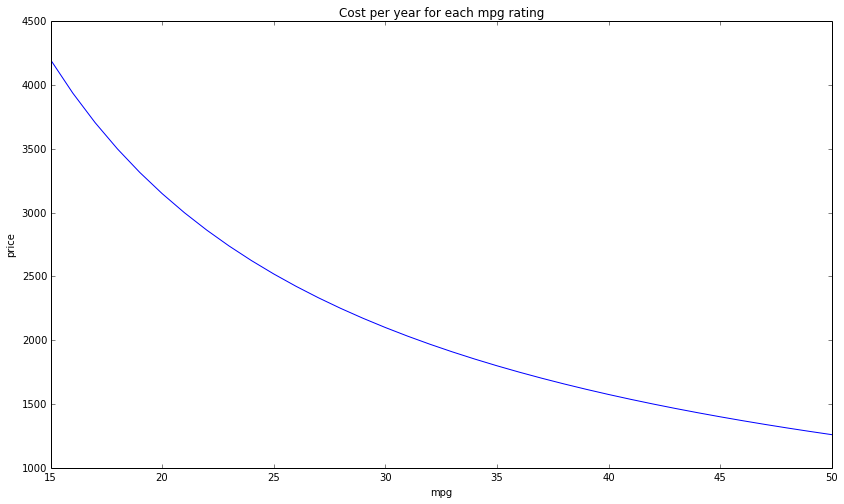# Fuel efficiency difference cost

I recently was car shopping, and I had the question about gas mileage: "How much does, say, the difference between 25 mpg and 30 mpg cost?"

To answer this question, I did a bit of analysis using IPython Notebook...

# Goal

• To find how much different fuel efficiencies cost in terms of dollars per year.
• I want to estimate this value for the next 10 or so years.

# Assumptions

• I'm estimating the average price per gallon of gas (over the next 10 years) to be $4.50. It is around$3.60 now, I adjusted for inflation, and counter-adjusted for the time-value of money.
• I'm estimating that we will drive about 14,000 miles per year (based on the last 3 years).

# Data

In :
price_per_gallon = 4.50 # dollars per gallon
miles_per_year = 14000.0 # miles per year


# Calculations

In :
def price_per_year(mpg):
return (miles_per_year / mpg) * price_per_gallon

In :
# Compare fuel efficiencies between 15 mpg and 50 mpg
mpg_list = range(15, 51)
price_list = [price_per_year(mpg) for mpg in mpg_list]

In :
def setup_graph(title='', x_label='', y_label='', fig_size=None):
fig = plt.figure()
if fig_size != None:
fig.set_size_inches(fig_size, fig_size)
ax.set_title(title)
ax.set_xlabel(x_label)
ax.set_ylabel(y_label)

setup_graph(title='Cost per year for each mpg rating', x_label='mpg', y_label='price', fig_size=(14,8))
_ = plot(mpg_list, price_list)# Example mpg differences in price¶

### 1 mpg difference (29 mpg to 30 mpg)¶

In :
price_per_year(29) - price_per_year(30)

Out:
72.41379310344837


### 5 mpg difference 20 mpg to 25 mpg)¶

In :
price_per_year(20) - price_per_year(25)

Out:
630.0


### 5 mpg difference (25 mpg to 30 mpg)¶

In :
price_per_year(25) - price_per_year(30)

Out:
420.0


### 5 mpg difference (30 mpg to 35 mpg)

##### Note the diminishing returns as fuel efficiency increases
In :
price_per_year(30) - price_per_year(35)

Out:
300.0


### 5 mpg difference (35 mpg to 40 mpg)¶

In :
price_per_year(35) - price_per_year(40)

Out:
225.0


### 10 mpg difference (20 mpg to 30 mpg)¶

In :
price_per_year(20) - price_per_year(30)

Out:
1050.0


### 10 mpg difference (25 mpg to 35 mpg)¶

In :
price_per_year(25) - price_per_year(35)

Out:
720.0


### 10 mpg difference (30 mpg to 40 mpg)¶

In :
price_per_year(30) - price_per_year(40)

Out:
525.0


### 10 mpg difference (40 mpg to 50 mpg)¶

In :
price_per_year(40) - price_per_year(50)

Out:
315.0